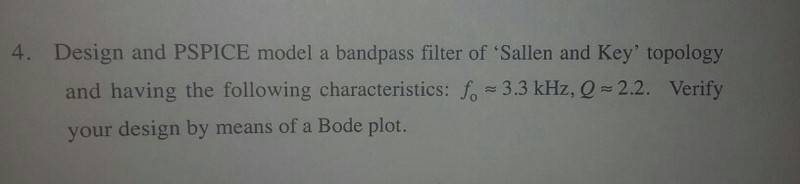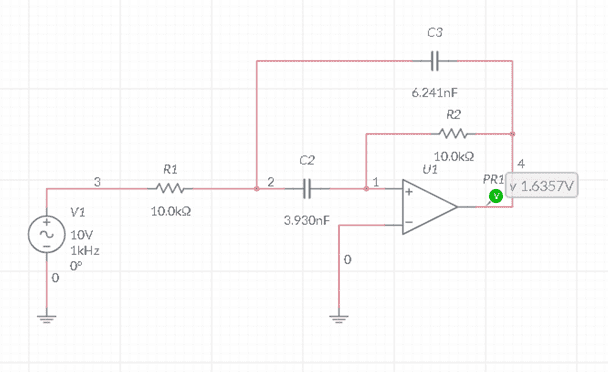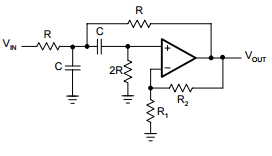# Sallen-Key Filter Homework: Tips to Improve Peak Voltage

• Ravrage
In summary, the author is looking for help with designing a bandpass filter. He has found the design equations and selected a circuit that is not a Sallen-Key design.f

## Homework Statement## The Attempt at a SolutionI worked out the Bandwidth at: 1500Hz so therefore Fh should be 4050Hz and Fl should be 2550Hz

Could someone give me any tips as to how correct my key is? with respect to the Bode plot on the sinusoidal output of my design the peak voltage is 2.2331 so the dB output of 20log(vo/vin) gives me 6.9782 which seems a little low.

Any help is much appreciated

#### Attachments

•NascentOxygen
Op amp inputs have been accidently swapped in your schematic, too.

•berkeman
Op amp inputs have been accidently swapped in your schematic, too.
That's a common problem using simulation software. Those of us that started more than a few decades ago learned that the "-" input was drawn towards the top and the "+" was at the bottom. At some point that standard was apparently declared 'inappropriate' and the simulation libraries swapped the inputs on all their op-amps. This not only confused the users but broke many simulations, and schematics, that were updated using the newer libraries. And of course the textbooks take a lot longer to update.

I still wonder about the rationale for this change. (and would dearly love to use something stronger than a wet noodle on whoever promulgated it)

•NascentOxygen
Op amp inputs have been accidently swapped in your schematic, too.
Actually, what he has drawn is a multiple feedback filter with the opamp inputs swapped. In a sallen key filter, the input is on the positive input and there is positive feedback with the negative input taken from a voltage divider on the output.

More than that - the shown circuit is not a lowpass but a BANDPASS filter in MFB topology.

More than that - the shown circuit is not a lowpass but a BANDPASS filter in MFB topology.
No one has suggested otherwise, surely?

Sorry, I was of the opinion (dont ask me why) that the question was "Sallen-Key lowpass".

Berkeman, this was the one I thought was the most appropriate as it is very briefly shown in my notes as a bandpss filter with no other explanations.

I got the bandwidth of 1.5kHz by dividing the fo/Q. this gives the upper and lower corner frequencies
I used the value of R1 as 3.3Kohm which using the equation R2=R1(2Q) squared = 62.888k ohm

Using the equation 1 / (2x pi x f x R) I got C1 as 6.241nF and C2 as 3.930nF so this is where th above design has come from.

But you're right the inputs of the op amp are the wrong way around

I'm just looking for a bit of help as to whether I'm heading in the right direction?

Thanks for the replies

Here are the design equations for your circuit:

Mid frequency: wo=(1/R1C2)*SQRT[R1C2/R2C3] ,
Midband Gain: Ao=R2/[R1*(1+C3/C2)]
Q-factor: Q=SQRT[Ao/(1+C2/C3)]=SQRT[R2C3/R1C2*(1+C3/C2)²]

I forgot to mention that a simple design allows two equal capacitors (C2=C3).
In this case, we have R2/R1=4Q².

I'm just looking for a bit of help as to whether I'm heading in the right direction?
Does it matter that the bandpass circuit you are considering is not a Sallen & Key design?

ermm that would be yes, damn.

ermm that would be yes, damn.
So - why didnt you show us the chosen circuit (because there are some alternatives) and the correspondiung questions?

Because that was my chosen design, looking online and at my notes I thought this was Sallen & key

Because that was my chosen design, looking online I thought this was Sallen & key
Yes - I understand. However, since several days you know that it it is not.
So - I suppose you have found Sallen-Key based realizations in the meantime.?
Which one did you select?

I'm not quite getting what you're saying? According to my notes (and reading back up) I can't see why this isn't a bandpass filter up until about an hour ago when NascentOxygen said it wasn't?

sorry now I see that you said MFB topology but I was on holiday and only had a chnace to look at it closely today

The shown circuit is a bandpass - however it does not use the so-called "Sallen-Key" topology (which is required according to the copied task description in your first post), That was the background of NascentOxygen`s remark.

Note that there are many different active circuit realizations for designing a bandpass - and two of them are Sallen-Key (with some alternatives) and Multi-feedback (MFB), which was shown by you in your first post. But - as far as I understand - you need the Sallen-Key.structure.
Did you start a search to find such a circuit ?

As soon as you show us the selected circuit we can give you some further help...(we cannot do all the work for you)

•Ravragewould be the one I would look at, sorry for wasting your time previously.

I'm not really asking for all the working to be done for me, merely an understanding of what I'm doing, apologies if it comes across that way

#### Attachments

OK - looks good.

You need an "understanding" of what You "are doing"?
OK - here is an explanation of the working principle:
As you probably know, the the first 4 elements form a passive bandpass (R-C-C-2R).
However, such a passive RC bandpass has a drawback - it can realize only filter Q values which are < 0.5 .
For larger Q values (smaller bandwidth) we add some positive feedback using a non-inverting amplifier (gain value determined by R1 and R2) and a feedback resistor R from the amplifier output to the first node of the input network.
All you have to do is to find the transfer function (classical methods for computing opamp gain characteristics with feedback) and to derive the formulas for designing the circuit according to the requirements.